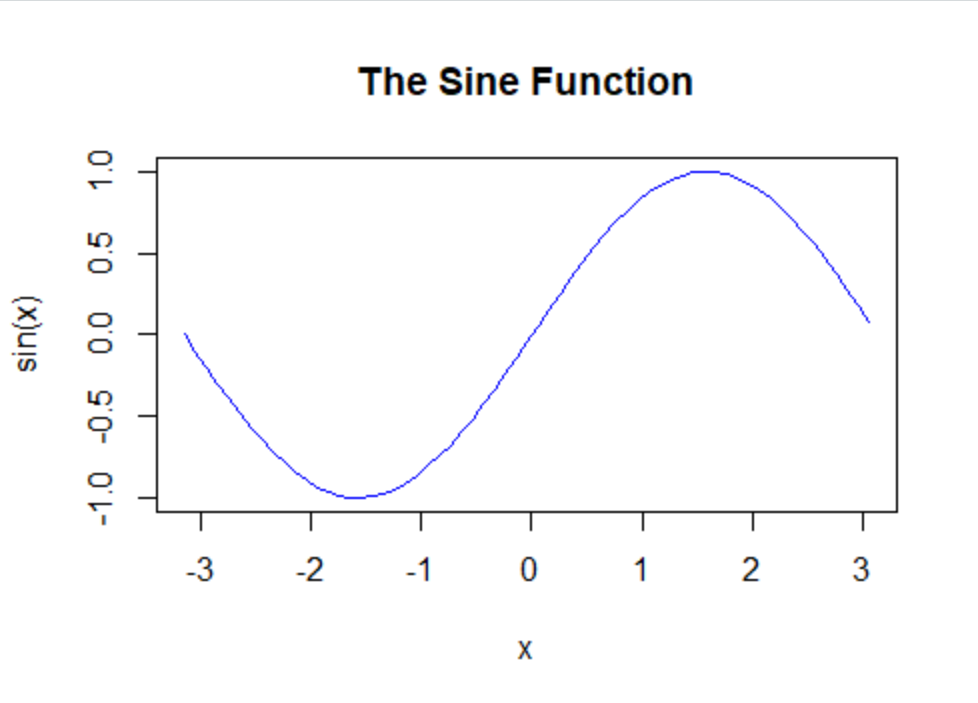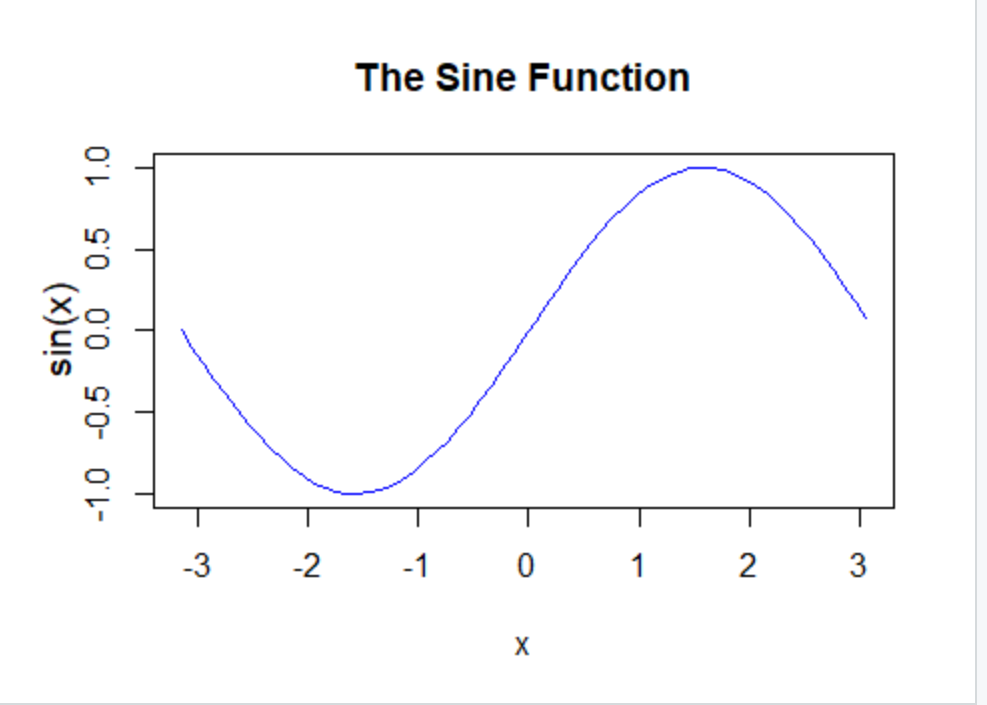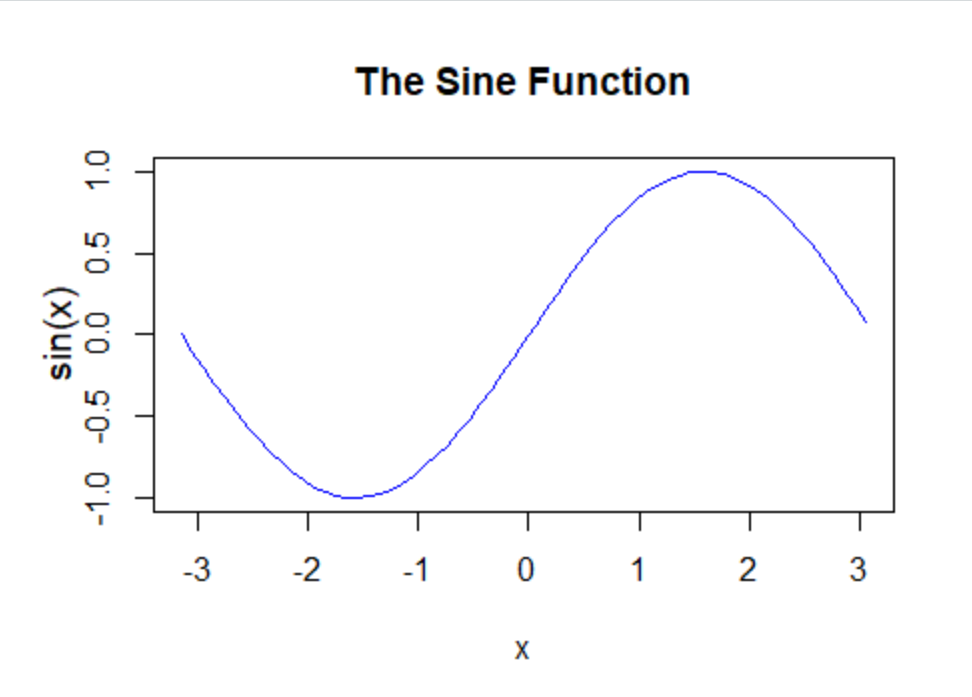Related Articles

# Move Axis Label Closer to Plot in Base R

• Last Updated : 29 Jun, 2021

In this article, we will discuss how to move the axis label closer to the plot in R Programming Language.

Before changing the distance, let us see how the initial plot will look like.

Example:

## R

 `x <- ``seq``(-``pi``,``pi``,0.1)`` ` `plot``(x, ``sin``(x),``    ``main=``"The Sine Function"``,``    ``ylab=``"sin(x)"``,``    ``type=``"l"``,``    ``col=``"blue"``)`

Output:Output 1- See the gap between sin(x) and y variable points

### Method 1: Using line argument

In the above example simply add the title() function with line argument. To move axis labels closer to the axis pass appropriate value to the line argument.

Example:

## R

 `x <- ``seq``(-``pi``,``pi``,0.1)`` ` `plot``(x, ``sin``(x),``    ``main=``"The Sine Function"``,``    ``ylab=``""``,``    ``type=``"l"``,``    ``col=``"blue"``)`` ` `title``(ylab=``"sin(x)"``, line=2, cex.lab=1.2)`

Output:Now check the difference!

### Method 2: Using mgp()

The second way to achieve the same desired output will be to adjust the mgp values. The default value for distance between axis and axis name is 3. Reduce the value to reduce the distance between them.

Syntax:

mgp(title, labels, line margin )

Parameters:

• title margin line for the axis title and its default value is 3.
• labels margin line for the axis labels and its default value is 1.
• Line margin value for the axis line and its default line is 0.

Example:

## R

 `x <- ``seq``(-``pi``,``pi``,0.1)`` ` `plot``(x, ``sin``(x),``    ``main=``"The Sine Function"``,``    ``ylab=``""``,``    ``type=``"l"``,``    ``col=``"blue"``)`` ` `title``(ylab=``"sin(x)"``, mgp=``c``(2,1,0),cex.lab=1.2)`

Output:Difference is same as line= 2.

My Personal Notes arrow_drop_up